[BACK]
 Intelligent Automation & Soft ComputingDOI:10.32604/iasc.2023.029644Article

Using GAN Neural Networks for Super-Resolution Reconstruction of Temperature Fields

1School of Artificial Intelligence, Nanjing University of Information Science & Technology, Nanjing, 210000, China
2Unit 93117 of PLA, Nanjing, 210000, China
3International Business Machines Corporation (IBM), New York, 100014, USA
4School of Computer and Software, Nanjing University of Information Science & Technology, Nanjing, 210000, China
*Corresponding Author: Zhiwei Jiang. Email: jzw0659@outlook.com
Received: 08 March 2022; Accepted: 21 April 2022

Abstract: A Generative Adversarial Neural (GAN) network is designed based on deep learning for the Super-Resolution (SR) reconstruction task of temperature fields (comparable to downscaling in the meteorological field), which is limited by the small number of ground stations and the sparse distribution of observations, resulting in a lack of fineness of data. To improve the network’s generalization performance, the residual structure, and batch normalization are used. Applying the nearest interpolation method to avoid over-smoothing of the climate element values instead of the conventional Bicubic interpolation in the computer vision field. Sub-pixel convolution is used instead of transposed convolution or interpolation methods for up-sampling to speed up network inference. The experimental dataset is the European Centre for Medium-Range Weather Forecasts Reanalysis v5 (ERA5) with a bidirectional resolution of 0.1×0.1 . On the other hand, the task aims to scale up the size by a factor of 8, which is rare compared to conventional methods. The comparison methods include traditional interpolation methods and a more widely used GAN-based network such as the SRGAN. The final experimental results show that the proposed scheme advances the performance of Root Mean Square Error (RMSE) by 37.25%, the Peak Signal-to-noise Ratio (PNSR) by 14.4%, and the Structural Similarity (SSIM) by 10.3% compared to the Bicubic Interpolation. For the traditional SRGAN network, a relatively obvious performance improvement is observed by experimental demonstration. Meanwhile, the GAN network can converge stably and reach the approximate Nash equilibrium for various initialization parameters to empirically illustrate the effectiveness of the method in the temperature fields.

Keywords: Super-resolution; deep learning; ERA5 dataset; GAN networks

1  Introduction

Artificial intelligence advances have shown considerable promise in the field of meteorology, not only in the domains of computer vision (CV) and language processing . The Image Super-Resolution  is a classic in the CV field; it generally refers to increasing the resolution, for example, from 512 × 512 to 1024 × 1024 pixels. In the field of meteorology, downscaling for the temperature field is a super-resolution reconstruction using the same notion as CV . It is a solution to mitigate the conversion of low-resolution data to high-resolution data. Most conventional statistical approaches employed in the past to develop statistical downscaling models in the meteorological disciplines were based on normal distribution assumptions. However, various extremes did not follow a normal distribution, and bottlenecks were encountered in the study of extreme climate events with non-normal distributions. .

GAN networks  use a random sample from the potential space as input and learn such that the output results are near to the true distribution in the training set, which overtakes the feature extraction performance of conventional neural networks. In this paper, In this research, we propose a GAN network structure and use the ERA5-Land hourly dataset [10,11] to scale up 2 m temperature field data with a resolution of 0.1×0.1 .

Overall, the contributions of this study are mainly in three aspects:

1.    To provide the model with adequate capacity and generalization performance, the widely used residual structure  and batch normalization  in the deep learning domain are included. On the other hand, a recurrent structure is required to fuse temporal information since the data are interrelated in time.

2.    Because arbitrary points in the image matrix have a defined physical relevance for meteorological elements such as temperature, using Bicubic interpolation for the scale down during the data processing phase, which will lose the original information, is ill-advised. In our work, the reduced location is perfectly aligned with the original image, and we use the nearest interpolation to retain the original image’s information.

3.    We adopt 2-fold staged zooming of the meteorological element field for the network’s structural attributes, which is more flexible with 2, 4, and 8-fold zooming. To enhance the GAN more effectively, sub-pixel  convolution is employed instead of the standard transposed convolution and interpolation approaches.

2  Related Work

Bilinear interpolation and Bicubic interpolation are extensively employed to recover lost information in images, whether it’s for image super-resolution or downscaling in the meteorological field. The basic idea is to use linear and cubic functions to interpolate pixel coordinates horizontally and vertically in a bidirectional way. In Fig. 1, the bilinear and bicubic approaches are presented. Bilinear and Bicubic gather the nearest 4 and 16 points surrounding the target point, respectively, and 1st-order and 3rd-order polynomials would be used to infer the value of the target pixel.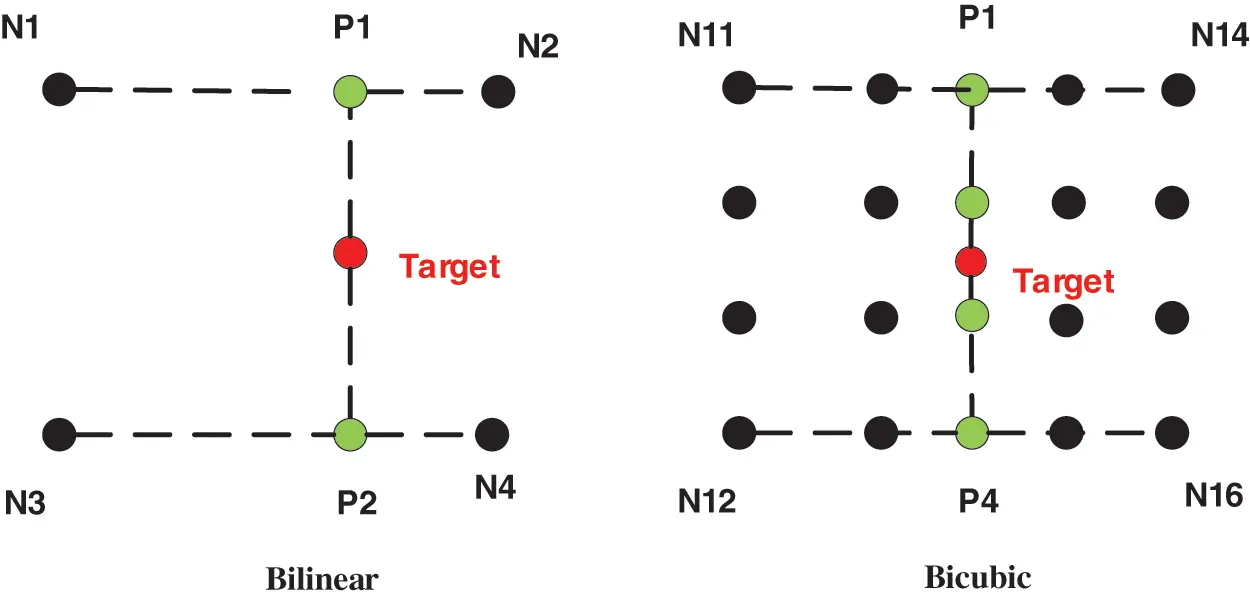Figure 1: Bilinear and Bicubic methods for target pixel values

The experimental results (Section 6) indicate that the interpolation method produces an overly smooth image. Deep learning approaches such as GAN-base, on the other hand, are preferred. GAN differs from ordinary neural networks in that it has two components: a generator and a discriminator. The generator’s job in the SR reconstruction task is to input a low-resolution picture and then generate a new high-resolution image, while the discriminator’s job is to distinguish between actual and fake images (by generator). It can approximate the produced data to the real distribution as the number of iterations increases. The GAN network’s CV field may be used in a variety of applications, including image style conversion and image demosaic.

Numerous works have been produced over the years with deep learning methods for SR reconstruction, Super-Resolution Deep Convolutional Networks (SRCNN)  introduced deep learning methods into the field of image Super-resolution for the first time using only three layers of convolution to achieve state-of-the-art (STOA) performance, and Faster SRCNN (FSRCNN) improved the SRCNN for many measures to promote network’s effectiveness. Very Deep Super-Resolution (VDSR)  allows the network to learn the residuals of the high-frequency part of the image and the performance is further improved. GAN networks were originally implemented for super-resolution to enhance realism via perceptual loss and adversarial loss, alleviating the problem of losing high-frequency details when using RMSE as a loss function in Super-Resolution GAN  (SRGAN, shown in Fig. 2).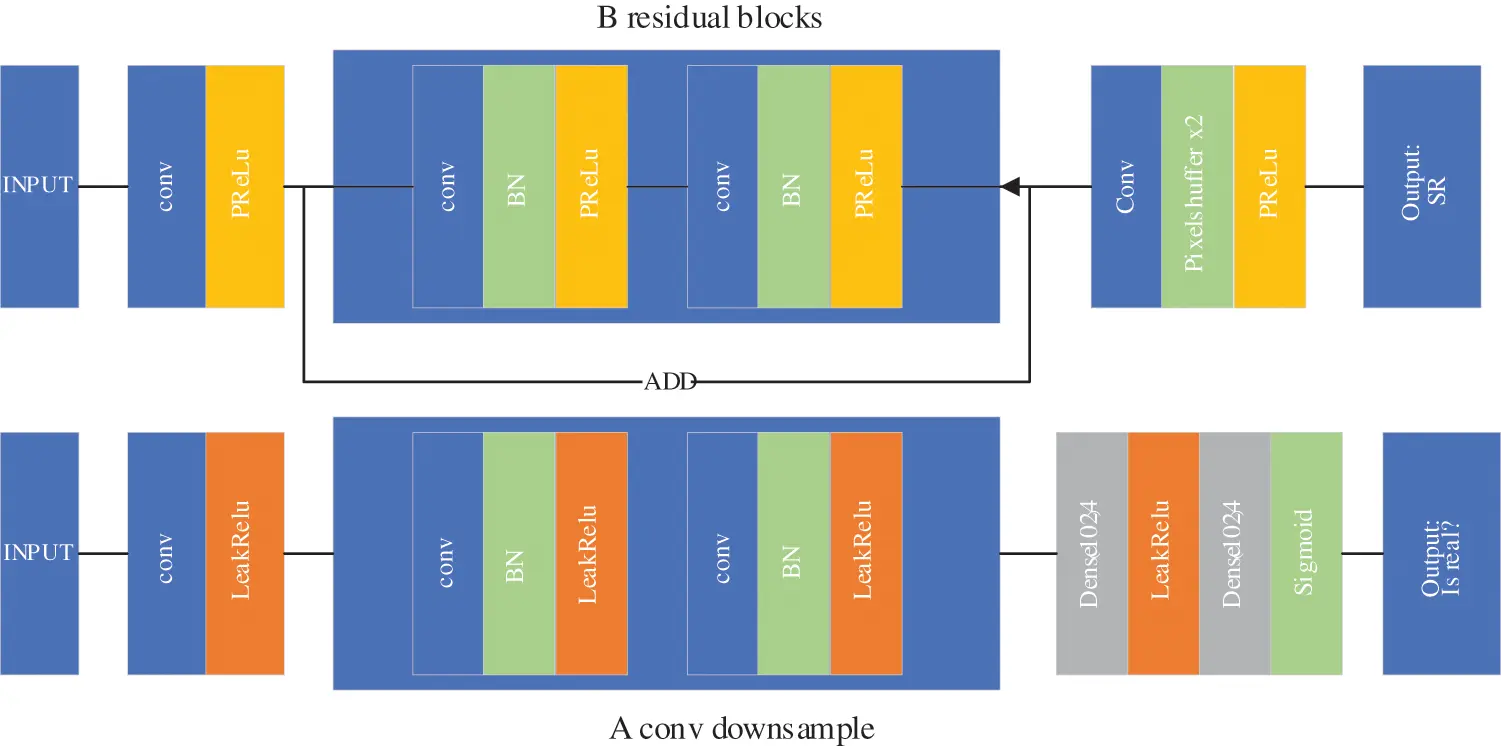Figure 2: SRGAN structure

The SRGAN needs high-resolution generation for all time frames by concatenating each frame due to the temporal correlation of the data, and we experimentally demonstrate that satisfactory results cannot be reached using the SRGAN approach for the temperature field SR reconstruction task, even though it is a baseline model in the picture super-resolution domain (results shown in Section 6).

Over the years Super-Resolution achievements in the non-computer vision domain have proliferated [18,19], and unlike image processing , it is critical to understand and quantify forecast uncertainty in climate and climate applications. The classical precipitation downscaling algorithm  uses techniques such as stochastic autoregressive models. Meteorology elements downscaling is also being attempted with deep learning. Leinonen et al.  used GAN networks for the down-sampling of rainfall and cloud thickness, and we differ from them by using pixel extraction in the down-sampling stage to retain the original information of meteorological elements while introducing sub-pixel convolution to optimize the network to be more efficient. The China Meteorological Administration’s Land Assimilation System Statistical Downscaling Model (CLDASSD)  used traditional convolution and proposed a quality control algorithm for high and low resolution data pairs, and achieved STOA performance for down-sampling the temperature field in the Chinese region.

3  Dataset

The experimental dataset is the ERA5-Land hourly , a reanalysis dataset with a higher resolution than ERA5 on pressure levels. The dataset uses physics laws to combine model data with observations from global regions into a dataset. The resolution of this dataset is 0.1° × 0.1°, one record per hour, and the meteorological element used is 2 m-temperature.

We crop the worldwide matrix for Beijing, China, with a longitude range of 111.2°E to 117.5°E and a latitude range of 36.0°N to 42.3°N., as shown in Fig. 3. The original dataset provided 00:00~23:00 with a total of 24 items for the temporal dimension, and we sampled the data at 3-h intervals to acquire 8 items in a day. After cropping and sampling the original data, the dimension shape in one day is D×T×1×H×W , where D is the number of days, T times per day ( T=8 in our experiment), the channel is 1 (one element), and H,W are the height and width, respectively.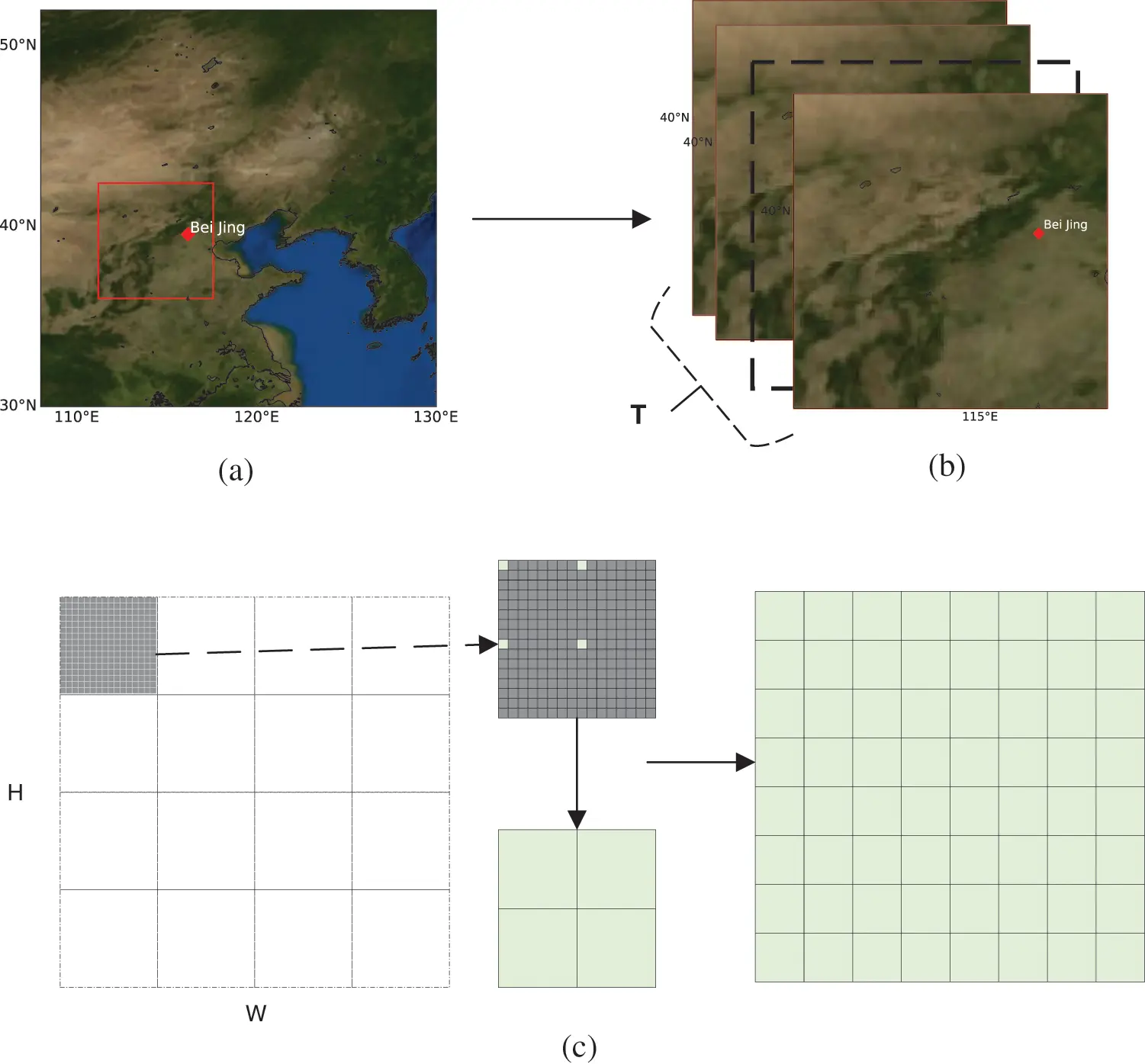Figure 3: ERA-Land data cropping and down-sampling

The original image needs to be downscaled to low-resolution and aligned with the original image for training, in addition to the concept of computer vision for SR. Bicubic interpolate is a generally used down-sampling method for converting a high-resolution image to a target resolution with a blurring effect, although it is ineffectual for meteorological data processing. To maintain the correct values of the real meteorological element field and prevent numerical smoothing, we adopt nearest interpolation down-sampling to upscale two points from the whole element field at k2 element points apart (except the boundary).

The training set contains all days for 2019 and 2020, the validation set is randomly divided from the training set to choose the best model during the training process, and the test set has 151 days of data from 2021.1.1 to 2021.5.31 to avoid temporal overlap with the training set.

4  Methodology

This experiment’s GAN is composed of a generator and a discriminator. The generator inputs low-resolution meteorological elements (2-D matrix), and the output can be dynamically adjusted to upscale to high-resolution element fields by factors of 2, 4, and 8 on demand. In this section, we show the model network structure for a generator and a discriminator, followed by an introduction to the network’s submodules such as residual structure, recurrent structure, and sub-pixel convolution.

The complete proposed GAN network structure is illustrated in Fig. 4. A generator is the main model of super-resolution for the low-resolution input and outputs of the high-resolution 2-D meteorological element field: the low-resolution part first input to the conventional convolution layer L1 , which expands 1 channel into large channels (256 in the experiment) and obtains data O1 , then O1 is summed with Gaussian noise N derive the latest O1 (the shapes O1 and N are equivalent).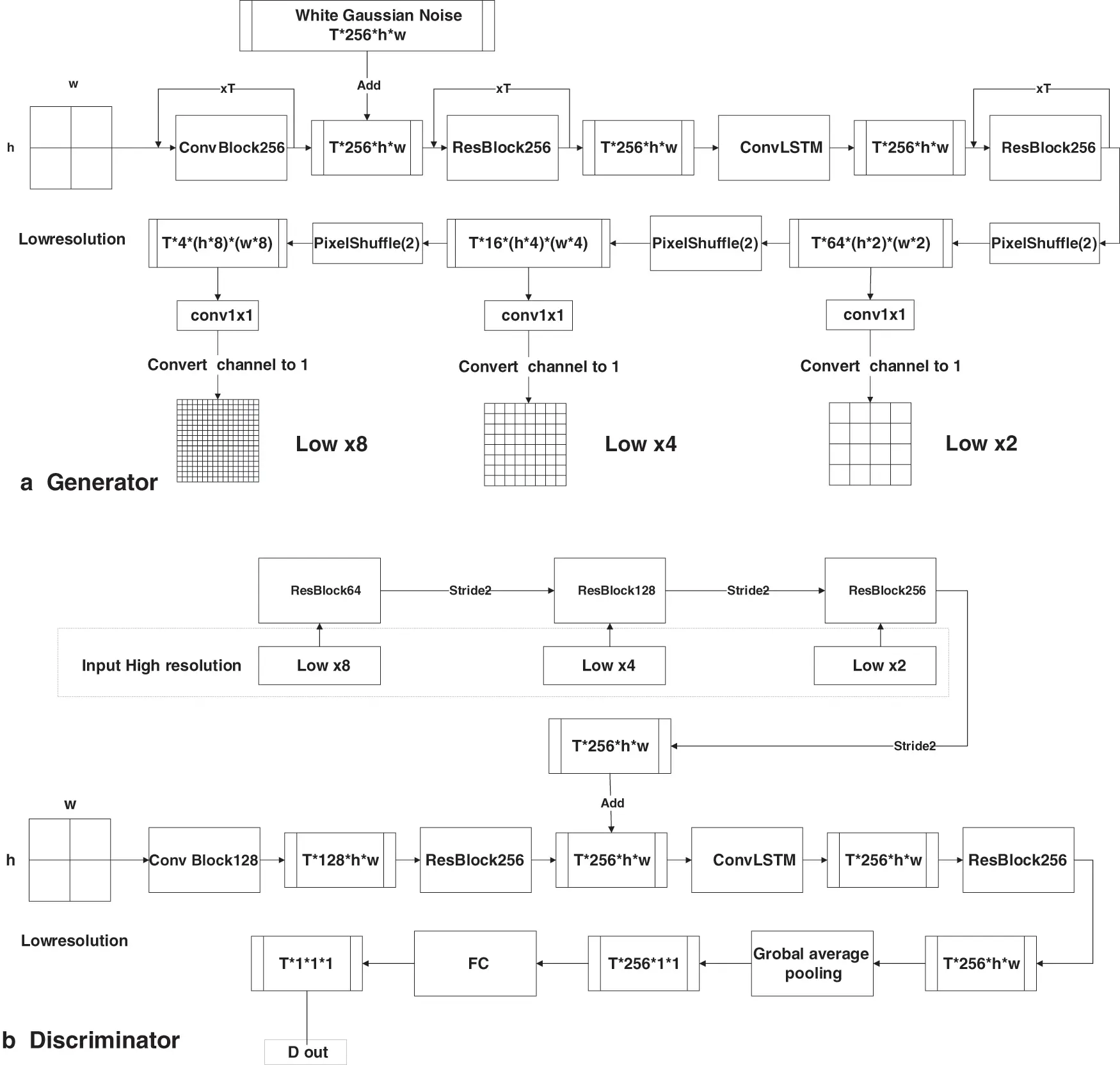Figure 4: Generator and Discriminator network overall structure

L2 consists of a residual structure that serves the same purpose as the convolutional layer, and is internally composed of multiple convolutional kernels, with residual connections tackling the issue of difficult learning after deepening the network.

The third layer of the generator L3 is the Convolutional Long Short Term Memory (ConvLSTM)  structure, which can be used for the temporal information of 2-D data and is a variant of the LSTM network for the 1-D time series prediction task, and can effectively solve the vanishing gradient problem in the timing network by migrating the gating structure into the convolutional layer. The structure L4 and L2 are the same as L5 ~ L7 , and sub-pixel convolution operations are used to provide progressive down-sampling (zooming he w and h in the image). The network layers before L5 are learnable layers, and the activation of the intermediate layer’s output is fixed in shape. L5 ~ L7 are to downscale the original image by a factor of 2 and implement flexible multiples of down-sampling on demand. The final layer is a 1 × 1 convolution used to convert the number of channels and yield the expected feature map output. The discriminator makes a judgment on the high-resolution images during the training process, it needs to input the low-resolution image over the high-resolution image, output a one-dimensional vector, and go through the sigmoid squeeze function to the [0,1] interval to represent the probability that the current input high-resolution image is the true result of the low-resolution image. Overall, the discriminator estimates the probability that a sample came from the training data rather than the generator.

Fig. 5 shows the submodules of ConvolutionBlock and ResBlock. ConvBaseBlock is an ordinary convolutional layer that fills normalizes, and non-linearly activates the input feature map (a fill operation is required before the input 3 × 3 convolutional layer so that the height and width of the image remain constant after convolution). ResBlock is the residual structure  that has arisen as a dominant structure in the CV domain, with gradient disappearance/explosion as network structure depth increases . The proposed paradigm solves the problem of network degradation, which speeds up the convergence of the training process, and drastically reduces the difficulty of training so that the network allows it to be designed deeper. The residual blocks process their inputs through two activation layers and convolutional layers, and finally, add the inputs to the outputs, replaces the traditional single convolutional layer with a single monolithic one, and the input and output can be achieved identically, while the network depth can be increased to improve the learning ability of the network. On the other hand, the Internal Covariate Shift (ICS) problem occurs as the network depth deepens, so we add batch normalization  after the output of the convolution layer and before the input of the activation function (except the last layer) can be used to solve it while allowing the activation function’s input data to fall in the gradient non-saturation region, mitigating the gradient disappearance risk and speeding up the training processes.Figure 5: GAN network submodules

In comparison to the conventional Bicubic interpolation, sub-pixel convolution  operates as shown in Fig. 6, which is a method for up-sampling distinguished from the interpolation function method by model-base. If the feature map needs to be scaled up twice, the number of channels will be expanded to yield 4 low-resolution images of the same shape by Convolutional Neural Network (CNN). The channel pixel sites can then be panned to the plane dimension to reduce the channel dimension in exchange for the plane scale.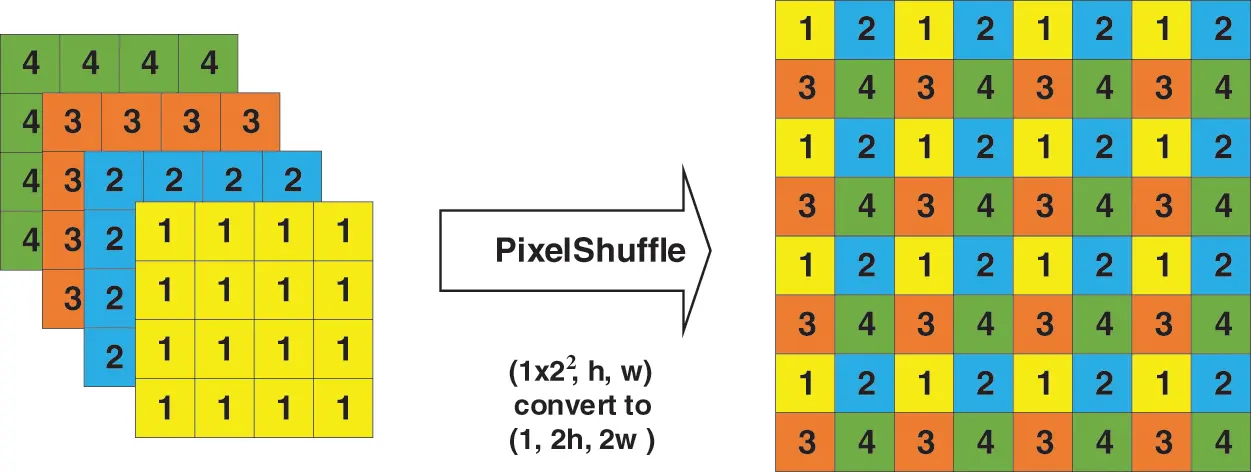Figure 6: Sub-pixel convolution (Pixel Shuffle)

5  Model Optimization Objectives

Our goal is to train a Generator ( G ) to generate realistic samples from random noise or latent variables, and a Discriminator ( D ) to discriminate between real and generated data, both of which are trained simultaneously until an approximate Nash equilibrium is reached, where the data generated by the Generator do not differ from the real samples and the Discriminator cannot correctly distinguish between the generated and real data, with the optimization objective as in Eq. (1), x denotes the real image, z denotes the input to G , z and n denote the input low-resolution image and Gaussian noise, respectively, and G(z) denotes the image generated by the G .

minGmaxDV(D,G)=ExPdata(x)[logD(x)]+E(z,n)Pdata(z,n)[log(1D(G(z,n)))] (1)

D() represents the probability of the D to judge whether the image is real or not, x refers to real image and the output of G(z) refers to a fake image. According to the objective function Eq. (1), the output of D(x) should be approximated to 1. D(G(z)) is the probability for D would judge the image generated by G (should be approximated to 0).

The role of G : as previously stated, D(G(z)) is the probability estimated by D that determines whether the image generated by G when z is the input, the optimization goal for G is to enable the generated image (output) to be as deceptive as possible to D . That is, G is to minimize V(D,G) such that the probability estimates of the output of D(G(z)) is approximated by 1. Comparable to D : the objective for D is to maximize V(G,D) , it requires the maximum distinction between real and generated images (fake). D and G have opposing objectives and confront each other. The final training stops at equilibrium and reaches an optimal state called Nash equilibrium.

We choose a gradient-based method to calculate the gradient of the network model’s weight parameters ( θg and θd ), and update the parameters by gradient descent or gradient ascent. For other high-order optimization methods (Newton-based methods and Quasi-Newton-based methods), assuming an n-dimensional optimization objective, the computational complexity of the single-round Newton methods is O(n3) , while the L-BFGS of the BFGS in the Quasi-Newton family are O(n2) , O(mn) , because the second-order and higher-order optimization methods are limited by computational effort, memory, and communication costs. However, in deep learning, the magnitude of optimization problem n is usually very large and has reached 107 magnitude in ALexNet in the early years. These high-order optimization methods lose their advantage in the field of in-depth learning when compared with gradient reduction with O(n) complexity (first-order optimization methods).

The training procedure optimizes the GAN network for the objective Eq. (1), the discriminator D and generator G should to be fixed separately, and gradients are calculated for the minimum batch mb .

1. When D is fixed, the output of D is a constant, and the gradient is calculated for G to see Eq. (2), and mb denotes the minimum one batch size.

θgVmb(G,D)=θg1mbi=1mblog(1D(G(z(i),ni)) (2)

2. When G is fixed, the gradient is calculated for D as described in Eq. (3).

θdVmb(G,D)=θd1mbi=1mb[logD(x(i))+log(1D(G(z(i),n(i)))] (3)

Optimizer: we choose stochastic gradient descent with 0.2 momenta (SGDM, gradient ascent is SGAM)  as the optimization method and update the method as follows. Although many variants of optimization algorithms with momentum such as Adaptive Moment Estimation (ADAM)  have been shown to converge faster and more efficiently in a large number of experiments, there is also experimental evidence that adaptive methods are detrimental to machine learning. Reddi et al.  found that Adam may not converge in some cases, and stochastic gradient descent (SGD)  or SGDM are still the dominant optimization methods.

The stochastic gradient descent update strategy with momentum is as follows:Strategy for updating the learning rate: we also hope to reduce the risk of the learning process into the saddle surface. In this experiment, we use a cosine annealing learning rate decay strategy to periodically update the learning rate. The following is how the learning rate update strategy works in Eq. (4), lr0 denotes the initial learning rate, etamin denotes the lower bound of learning rate, Tmax is the number of iterations required for one cosine cycle, epochi denotes the location of the current iteration, LRsch denotes the standard cosine annealing strategy, and LRwarm represents epochi resets the learning rate after reaching the Tmax period, which makes the network converge rapidly, and the learning rate is smaller in the late training period, which makes the network converge to the optimal solution better. better converge to the optimal solution.

LRsch(epochi,Tmax,etamin)=etamin+0.5(lr0etamin)(1+cos(epochTmax))LRwarm(epochi,Tmax,etamin)={lr0ifepochi=TmaxLRsch(epochi,Tmax,etamin)ifepochiTmax (4)

The complete update process algorithm is as follows: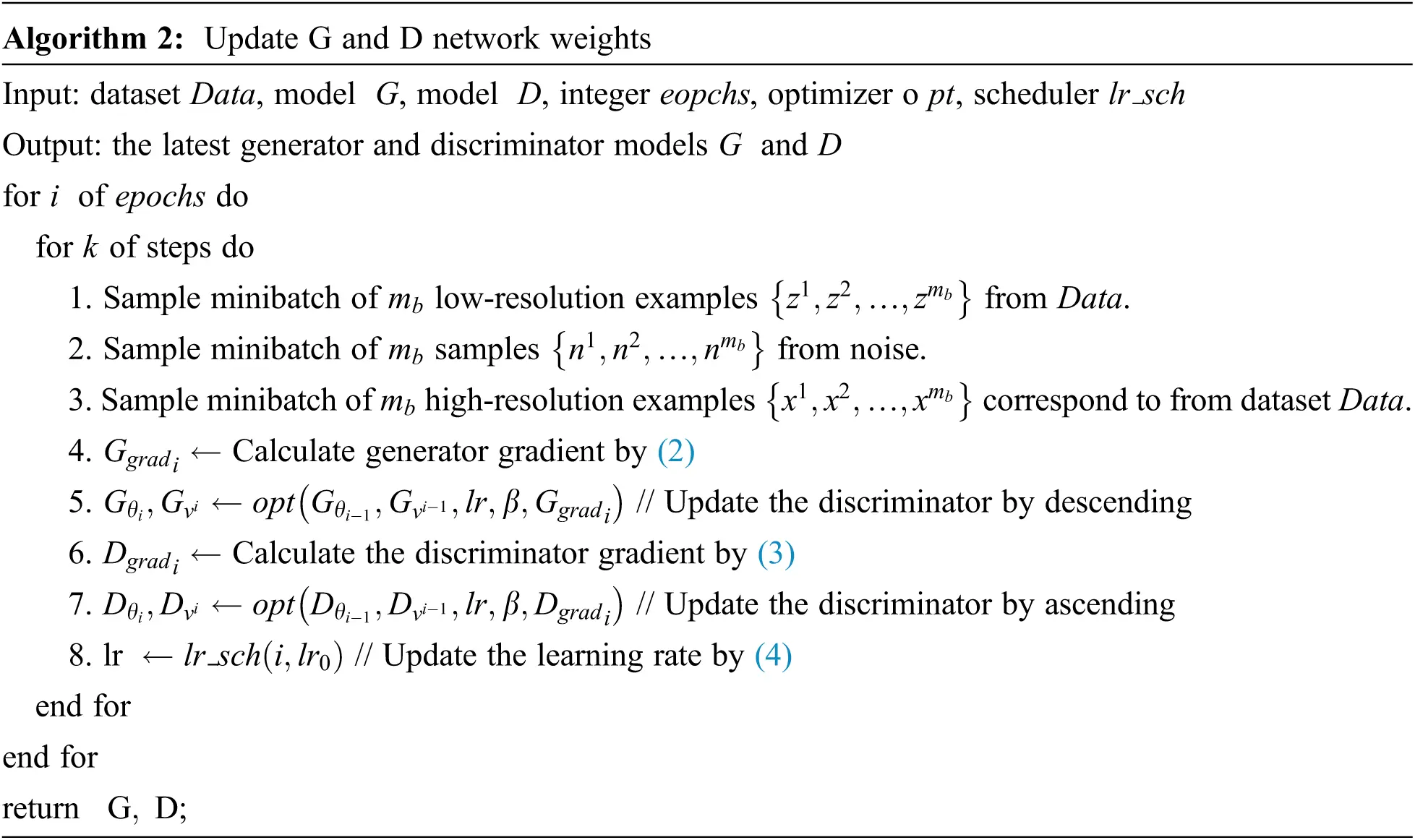5.1 Metrics

In this section, evaluation metrics widely used in the CV field are introduced and applied to the downscaled evaluation results of the meteorological element field in this paper. MSE is the most commonly adopted in machine learning as shown in Eq. (5), which indicates the error square of the current generated image G(z) and the reference image x . The smaller the MSE , the smaller the Euclidean distance between the generated image and the real image.

MSE=1mbi=0mbG(z(i),n(i))x(i)2T1WH (5)

For tasks with super-resolution, the mean square error has limitations, and it has been shown that using the root mean square error as the primary loss function loses high-frequency information from the image. The Peak Signal-to-noise Ratio (PSNR, shown by Eq. (6)) is the ratio between the maximum power of the signal and the signal noise power to measure the quality of the reconstructed image that has been compressed, usually expressed in decibels (dB), the higher the PSNR index, the better the image quality, MAXI2 represents the maximum value, which is set to 255 in RGB images, while for meteorological elements, we will set the element field according to the history to 50.

PSNR=10log10MAXI2MSE (6)

MSE, PSNR is not consistent with the actual visual perception of human eyes, we also applied Structural Similarity (SSIM)  as the evaluation metric, the SSIM algorithm is designed to consider the visual characteristics of human eyes, which is more consistent with the visual perception of human eyes than the traditional way, it is a measure of the similarity of two images, and the value range is [0,1], the larger the value of SSIM, it means less distortion or better quality of the image. The higher SSIM, the less distorted the better quality. SSIM is calculated as in Eq. (7), where μ denotes the image mean, σ denotes the covariance, and C is a constant to avoid numerical crashes.

Zout=G(Z,N)L(Zout,X)=2μzμx+C1μz2+μx2+C1C(Zout,X)=2σzσx+C2σz2+σx2+C2S(Zout,X)=σzx+C3σzσx+C3SSIM(Zout,X)=L(Zout,X)×C(Zout,X)×S(Zout,X) (7)

5.2 Convergence Verification

We verified the convergence of the GAN by empirical methods, observing the average metric performance of the output of the generator G to the real labels during the training process, and for the output of the discriminator, considering the proof of  for the convergence of Nash equilibrium in GAN networks, we calculated D(X,Zout) at each step of the training process, with the formula shown in Eq. (8), assuming that the image produced by Zout is exactly the same as the real image X , at this time, D(X,Zout)=0.5 , which indicates that the discriminator cannot recognize the image as true or false, and the whole network is in Nash equilibrium and cannot continue learning. The details of the training process and the experimental description of the convergence point of the Nash equilibrium are described in 6.2.

Zout=G(Z,N)D(X,Zout)=D(X)D(X)+D(Zout) (8)

6  Evaluation

6.1 Experimental Results

In this section, we compare the performance of interpolation methods (Bilinear, Bicubic) and GAN network methods on the test dataset (date from 2021-1-1 to 2021-5-31). To evaluate the generalization performance of our method with different parameters, we applied different T0 settings for the cosine annealing learning rate decay strategy and fixed other parameters including hyperparameters (such as the model initialization weight and learning rate) to demonstrate the results. As the evaluation results on the test set are shown in Fig. 7, the performance of the proposed model at T0=300 setting for all metrics performs better than the settings T0=150 and T0=100 . From a seasonal prespective, the average performance of the GAN network outperforms the interpolation methods between January and February, and the GAN network’s advantage becomes more apparent as days pass. We also include a comparison of SRGAN on the same test set, and the results on the test set show that our proposed model has improved performance in MSE, SSIM, and PSNR metrics for the majority of dates, which verifies the validity of the proposed model structure compared to GAN-based model.Figure 7: Comparison of MSE, PSNR, and SSIM performance of GAN-based and interpolation methods

We sampled 3 days of the test dataset (Date: 1–1, 3–7, 5–31) to represent the results of the Pre-, mid-and-late period, respectively, and the actual performance of downscaling is shown in Fig. 8, we can see that in the Pre-period, the proposed model is visually insignificantly distinct from other methods, still has slightly better performance. As the days increase, the advantages of our GAN model gradually apparent, capable of restoring more detailes.Figure 8: Test set sampling results performance

6.2 Training Details

We fix the parameters of the optimizer and the network weights’ initial except the learning rate decay strategy, using the SGD optimizer with 0.2 momenta, the initial learning rate is 0.005, and the number of iterations is set to 300. The performance of each metric during the training process is recorded as shown in Fig. 9 and the result is shown in Tab. 1, where the learning rate is represented by the last line, and the others represent MSE, PSNR, SSIM and D(X,Zout) , respectively. It can be seen that the neural network is more sensitive to different T0, and when the learning rate is reset in the middle of training the training will show large fluctuations, for example, T0=150 and T0=100 . The MSE blank indicates that exceed 100 is not shown in Fig, and the PSNR is at a low level in the blank interval. For the verification of convergence, the value of D(X,Zout) can eventually converge approximately 0.5 as the number of training increases for different T0 . Tab. 1 shows the overall performance of the different models and SRGAN in the test set. The results show that the performance of the model proposed in this paper is better than that of the interpolation method and SRGAN.Figure 9: The performance of each index during the training process for different T07  Conclusion

GAN is one of the most prominent deep learning approaches and has made significant progress in image and video super-resolution. The enhancement of resolution has wide applicability in observation and model data processing in climate science. This work addresses the growing demand by generating a conditional super-resolution GAN that operates on a 2-dimensional image sequence for each input. Rather than processing each image independently in a sequence, our generator and discriminator structures develop the concept of recurrent neural networks to apply to temporal data, the results demonstrate that GAN network-based models generally outperform traditional interpolation methods, while our proposed GAN network performs better than the ordinary GAN-based model.

The proposed model also has limitations. Since it is a GAN-based model, the limitations that exist in GAN networks are also potential threats to our model: (1) Model parameters oscillate, falter, and do not converge, although it did not appear in our experiments, it is still something that should be taken seriously. (2) The complexity of the model is higher compared to SRGAN, and the training and inference speed is not advantageous compared to it. (3) It is limited by the complexity of the model, which requires sufficient time or rounds to adequately converge. (4) The current magnification is not flexible enough; it hopes to be able to further expand in more applications.

1.    Optimization of the network structure to improve performance and memory usage.

2.    Generalization of different scale factors, producing high-resolution images with multiple scale factors at once (the current version is specific to a factor of 8), although it is possible to switch flexibly between 2x or 4x, all require the support of the dataset and retraining, while for the output is not able to output multiple for once time.

3.    It is preferable to implement frame insertion in the temporal dimension in addition to the spatial dimension.

4.    Extrapolation of time series to allow short-term prediction for prospects.

5.    Employing auxiliary variables may prompt the output of the generator to approximate the real distribution better. For example, the altitude is input to our network as an auxiliary variable for fusing more meteorological-related information.

Funding Statement: This research was supported by the National Natural Science Foundation of China under Grant Nos.61772280 and 62072249.

Conflicts of Interest: The authors declare that they have no conflicts of interest to report regarding the present study.

## References

1. C. Liu, S. Yang, D. Di, Y. Yang, C. Zhou et al., “A machine learning-based cloud detection algorithm for the himawari-8 spectral image,” Advances in Atmospheric Sciences, vol. 38, pp. 1–14, 202
2. H. Li, C. Yu, J. Xia, Y. Wang, J. Zhu et al., “A model output machine learning method for grid temperature forecasts in the Beijing area,” Advances in Atmospheric Sciences, vol. 36, no. 10, pp. 1156–1170, 2019.
3. H. Dai, “Machine learning of weather forecasting rules from large meteorological data bases,” Advances in Atmospheric Sciences, vol. 13, no. 4, pp. 471–488, 1996.
4. J. Xia, H. Li, Y. Kang, C. Yu, L. Ji et al., “Machine learning−based weather support for the 2022 winter olympics,” Advances in Atmospheric Sciences, vol. 37, no. 9, pp. 927–932, 2020.
5. L. Han, M. Chen, K. Chen, H. Chen, Y. Zhang et al., “A deep learning method for bias correction of ECMWF 24–240 h forecasts,” Advances in Atmospheric Sciences, vol. 38, no. 9, pp. 1444–1459, 2021.
6. I. Michal and P. Shmuel, “Improving resolution by image registration,” GVGIP : Graphical Models and Image Processing, vol. 53, no. 3, pp. 231–239, 1991.
7. B. C. Hewitson and R. G. Crane, “Climate downscaling: Techniques and application,” Climate Research, vol. 07, no. 2, pp. 85–95, 1996.
8. C. Qian, W. Zhou, S. K. Fong and K. C. Leong, “Two approaches for statistical prediction of non-gaussian climate extremes: A case study of macao hot extremes during 1912–2012,” Journal of Climate, vol. 28, no. 2, pp. 623–636, 2015.
9. I. Goodfellow, J. Pouget-Abadie, M. Mirza, B. Xu, D. Warde-Farley et al., “Generative Adversarial Nets,” in Advances in Neural Information Processing Systems 27th, Montreal, Quebec, Canada, pp. 2672–2680, 2014.
10. H. Hersbach, B. Bell, P. Berrisford, S. Hirahara, A. Horányi et al., “The ERA5 global reanalysis,” Quarterly Journal of the Royal Meteorological Society, vol. 146, no. 730, pp. 1999–2049, 2020.
11. J. Muñoz Sabater, “Copernicus climate change service (C3Sclimate data store (CDSERA5-land hourly data from 1981 to present,” 2019. [Online]. Available: http://dx.doi.org/10.24381/cds.e2161bac.
12. K. He, X. Zhang, S. Ren and J. Sun, “Deep residual learning for image recognition,” in IEEE Conf. on Computer Vision and Pattern Recognition (CVPR), Las Vegas, NV, USA, pp. 770–778, 2016.
13. S. Ioffe and C. Szegedy, “Batch normalization: Accelerating deep network training by reducing internal covariate shift,” in Proc. of the 32nd Int. Conf. on Machine Learning, Proc. of Machine Learning Research, Lille, France, pp. 448–456, 2015.
14. W. Shi, J. Caballero, F. Huszár, J. Totz and Z. Wang, “Real-time single image and video super-resolution using an efficient sub-pixel convolutional neural network,” in 2016 IEEE Conf. on Computer Vision and Pattern Recognition (CVPR), Las Vegas, NV, USA, pp. 1874–1883, 2016.
15. C. Dong, C. C. Loy, K. He and X. Tang, “Image super-resolution using deep convolutional networks,” IEEE Transactions on Pattern Analysis and Machine Intelligence, vol. 38, no. 2, pp. 295–307, 2016.
16. J. Kim, J. K. Lee and K. M. Lee, “Accurate image super-resolution using very deep convolutional networks,” in 2016 IEEE Conf. on Computer Vision and Pattern Recognition (CVPR), Las Vegas, NV, USA, pp. 1646–1654, 20
17. C. Ledig, L. Theis, F. Huszar, J. Caballero, A. Cunningham et al., “Photo-realistic single image super-resolution using a generative adversarial network,” in IEEE Conf. on Computer Vision and Pattern Recognition (CVPR), Honolulu, HI, USA, pp. 105–114, 20
18. K. C. Aswathy and E. Poovammal, “A novel alphaSRGAN for underwater image super resolution,” Computers, Materials & Continua, vol. 69, no. 2, pp. 1537–1552, 2021.
19. K. Sathya and M. Rajalakshmi, “CNN: Enhanced super resolution method for rice plant disease classification,” Computer Systems Science and Engineering, vol. 42, no. 1, pp. 33–47, 2022.
20. W. El-Shafai, A.-M. Ali, E.-S.-M. El-Rabaie, N.-F. Soliman, A.-D. Algarni et al., “Automated COVID-19 detection based on single-image super-resolution and CNN models,” Computers, Materials & Continua, vol. 70, no. 1, pp. 1141–1157, 2022.
21. X. Liu, Z. Chen, W. Song, F. Li and Y. Yang, “Data matching of solar images super-resolution based on deep learning,” Computers, Materials & Continua, vol. 68, no. 3, pp. 4017–4029, 20
22. J. Zhou, J. Liu, J. Li, M. Huang, J. Cheng et al., “Mixed attention densely residual network for single image super-resolution,” Computer Systems Science and Engineering, vol. 39, no. 1, pp. 133–146, 2021.
23. N. Rebora, L. Ferraris, J. V. HarDeNberg and A. Provenzale, “RainFARM: Rainfall downscaling by a filtered autoregressive model,” Journal of Hydrometeorology, vol. 7, no. 4, pp. 724–738, 2006.
24. J. Leinonen, D. Nerini and A. Berne, “Stochastic super-resolution for downscaling time-evolving atmospheric fields with a generative adversarial network,” IEEE Transactions on Geoscience and Remote Sensing, vol. 59, no. 9, pp. 7211–7223, 2021.
25. R. Tie, C. Shi, G. Wan, X. Hu, L. Kang et al., “CLDASSD: Reconstructing fine textures of the temperature field using super-resolution technology,” Advances in Atmospheric Sciences, vol. 38, pp. 1–14, 2021.
26. X. Shi, Z. Chen, H. Wang, D.-Y. Yeung, W.-K. Wong et al., “Convolutional LSTM network: A machine learning approach for precipitation nowcasting,” Advances in Neural Information Processing Systems, vol. 28, pp. 802–810, 2015.
27. Y. Bengio, P. Y. Simard and P. Frasconi, “Learning long-term dependencies with gradient descent is difficult,” IEEE Transactions on Neural Networks, vol. 5, no. 2, pp. 157–166, 1994.
28. I. Sutskever, J. Martens, G. E. Dahl and G. E. Hinton, “On the importance of initialization and momentum in deep learning,” in Proc. of the 30th Int. Conf. on Machine Learning, Proc. of Machine Learning Research, Atlanta, GA, USA, pp. 1139–1147, 2013.
29. D. P. Kingma and J. Ba, “Adam: A method for stochastic optimization,” 2014. [Online]. Available: https://arxiv.org/abs/1412.6980.
30. S. J. Reddi, S. Kale and S. Kumar, “On the convergence of adam and beyond,” 2019. [Online]. Available: https://arxiv.org/abs/1904.09237.
31. H. Robbins and S. Monro, “A stochastic approximation method,” The Annals of Mathematical Statistics, vol. 22, no. 3, pp. 400–407, 1951.
32. Z. Wang, A. C. Bovik, H. R. Sheikh and E. P. Simoncelli, “Image quality assessment: From error visibility to structural similarity,” IEEE Transactions on Image Processing, vol. 13, no. 4, pp. 600–612, 2004.This work is licensed under a Creative Commons Attribution 4.0 International License, which permits unrestricted use, distribution, and reproduction in any medium, provided the original work is properly cited.